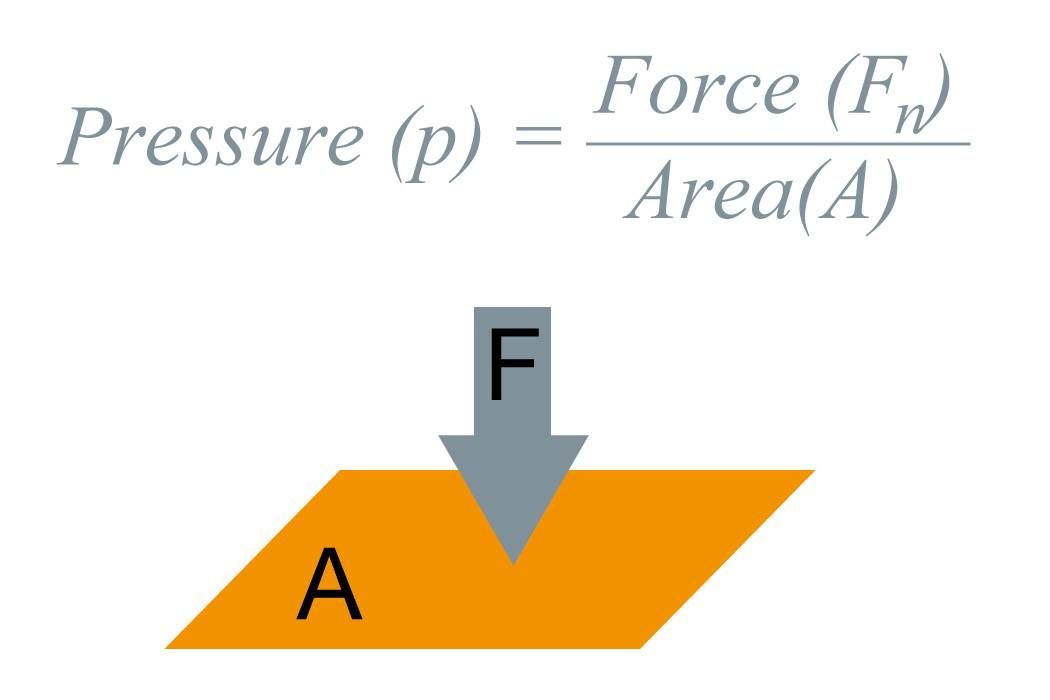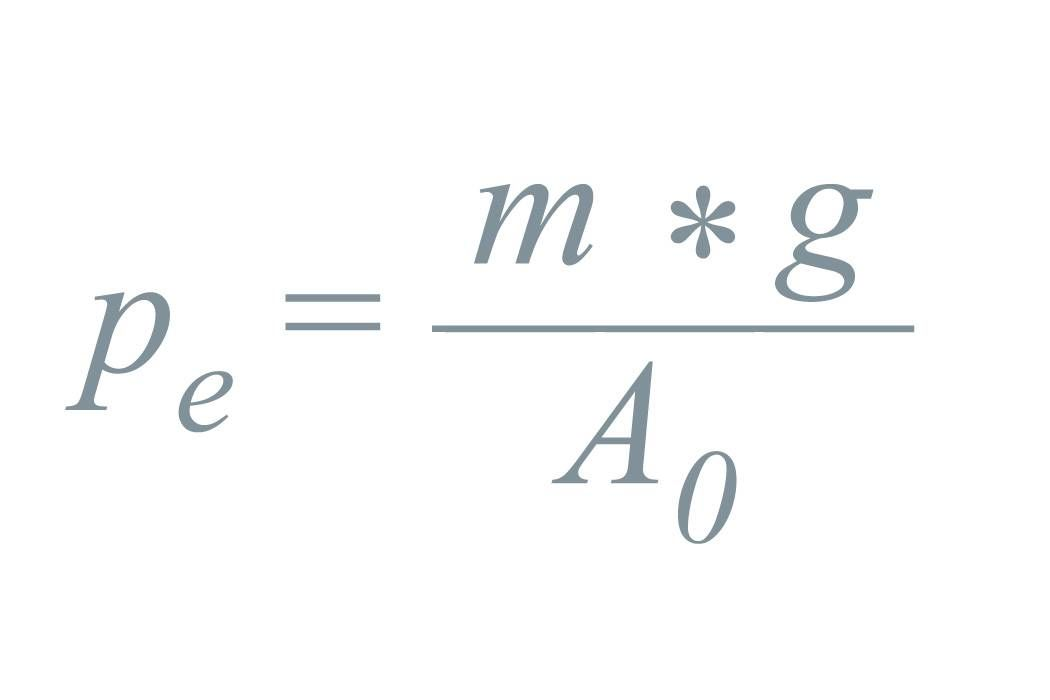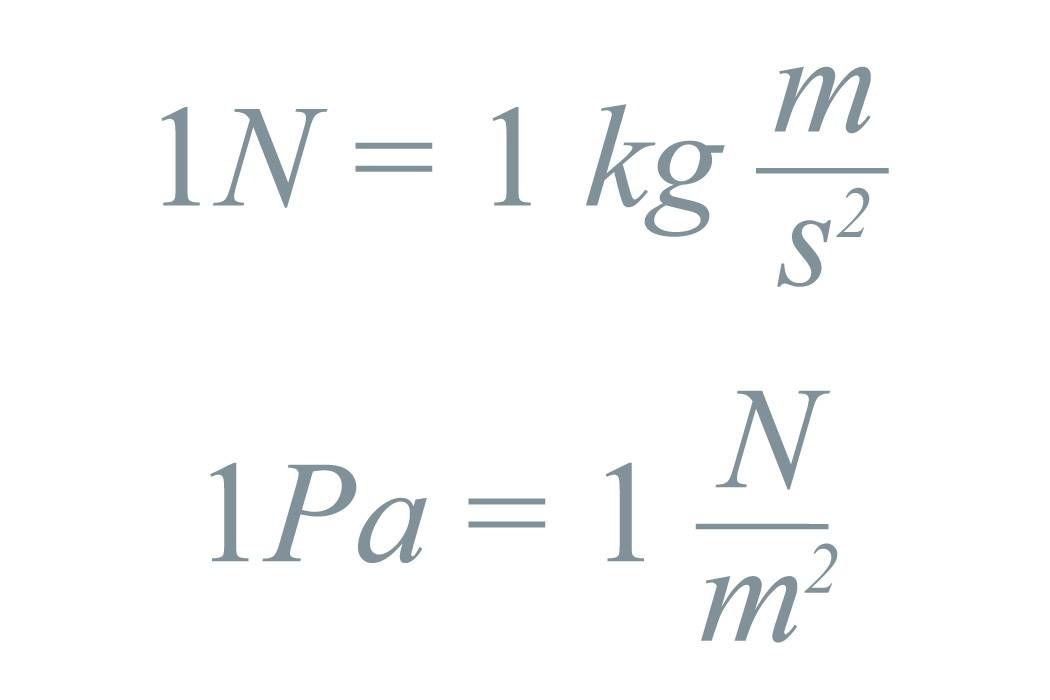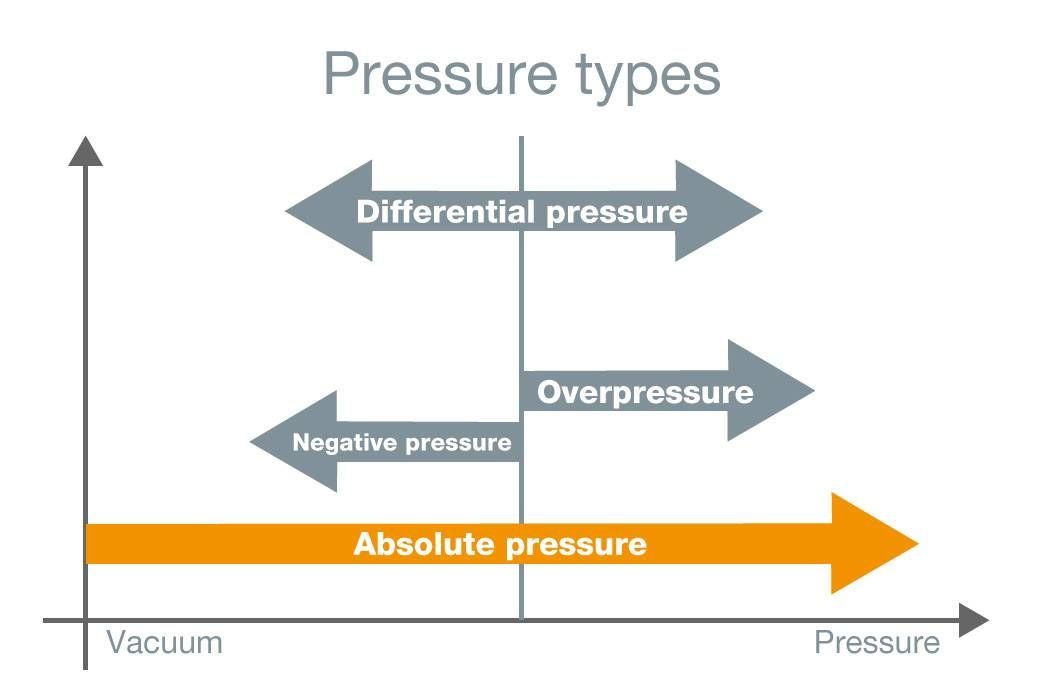Physical principlesWhat does pressure actually mean in the physical sense?

The state variable pressure (p) is defined as a force (Fn) which is uniformly applied to a certain surface (A). This force can be applied by a liquid, by gases or by solid bodies.Example pressure compensator

Pressure corresponds to the weight of calibrated weights according to the adjacent formula. p(e) = overpressure, m = mass of the weights, g = acceleration of gravity and A(0) = (effective) cross-section.The pressure units

The pressure unit Pascal (Pa) is derived from the unit of the (weight) force Newton (N), i.e. N je m². One pascal (Pa) corresponds to the pressure acting evenly on a surface when a force of 1 N is exerted vertically on an area of 1 m².

Pascal (Pa)

Measurement in cleanrooms or in flow velicity measurements with Pitot tube.

hPa (= mbar)

Gas flow pressure, gas static pressure

bar, KPa, MPa

Standard units in industrial pressure measuring technology.

mmHg

(= millimeters of mercury) Standard for medical technology (blood pressure) instead of the previous mmH2O.

micron

Smallest unit (750 micron = 1hPa) in the pressure range, e.g. when evacuating refrigeration systems.

Pressure types

Pressure measurement compares an actual pressure with a reference pressure. Pressure measuring technology distinguishes between the following types of pressure, enabling a statement to be made about the relationship between the measuring pressure and the reference pressure. Absolute pressure relates to the vacuous space of the universe (zero pressure).

Absolute pressure

• Measured pressure above absolute zero
• Reference, ideal vacuum
• Measuring pressure is always greater than reference pressure

Differential pressure

• Measured pressure above or below any desired reference pressure
• Measuring pressure is less or greater than reference pressurePositive pressure

• Measured pressure above the barometric daily air pressure
• Reference ambient pressure
• Measuring pressure is always greater than reference pressure

Negative pressure

• Measured pressure below the barometric daily air pressure
• Reference ambient pressure
• Measuring pressure is always less than reference pressure

Crucial to life: atmospheric pressure

• Atmospheric pressure arises through the weight of the atmosphere that surrounds the earth.